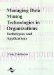# Introduction

 < Day Day Up >

This research considers the aggregation of expert point estimates, especially forecasts, under the assumption that each of these is an estimate of the minimizer, x* of an unknown loss function. Noting that the statistcal mode may be regarded as the maximizer of the density function, the question arises as to how the two problems might be related. By specifying some general properties of the loss function, l(x) and using a performance density, g(l) for the experts, a density, f(x) for the observed expert estimates can then be determined. Some sufficient conditions are then derived for the problem of determining when the mode of this density coincides with x*, the minimizer of l(x). Thus we obtain some results relevant to the question of the sufficiency of consensus for accuracy. Along with other considerations, these results suggest that the statistical mode can be expected to provide an effective method for aggregation of individual forecasts, when these are regarded as expert estimates of the point that minimizes forecast loss, i.e., the ideal point.

Intuition for these results arises first because the mode is a measure of central tendency. The mode must agree with the mean combination of forecasts when the distribution of estimates is symmetric. However, the mode should outperform the mean whenever that distribution is skewed. Next, for conditions under which the mode of the expert estimates density coincides with the ideal point, selection of the mode as estimate is a simple maximum likelihood decision.

A further source of intuition for these results is the notion that nearness of expert estimates may be indicative of nearness of these estimates themselves to the ideal point. Nearness of point estimates is thus conceived as an approximation to unanimity, which can not be achieved with positive probability. The point at which such closeness is greatest should be the mode of the distribution.

We also introduce the use of Markov chain Monte Carlo (MCMC) method in mode estimation. Using the Bayesian approach and the MCMC method, an empirical distribution corresponding to the predictive density of the expert estimates can be constructed. Hence the mode can be estimated and a probability interval for the forecast value can also be obtained. So far as we could determine the probability interval associated with a combination forecast has not been studied previously in the literature. Perhaps this is because in most cases one only has a few individual forecasts; thus, the estimation of the standard error of the relevant estimators will be based on a very small sample. This causes difficulty for standard estimation techniques, which are usually justified by asymptotic properties.

The chapter is organized as follows. "Model Development and Sufficiency Results" develops sufficient conditions which assure that the mode of the expert estimates (forecasting methods) is the point of smallest loss. "Individual and Combination Forecasts" outlines the ideas of forecast combination and introduces several methods that are used in our empirical example. "Bayesian Approach" is a brief introduction to the Bayesian approach, whereas "Markov Chain Monte Carlo Techniques" and "Log-Concavity Conditions" elaborate the Markov chain Monte Carlo technique used in the estimation. "Simulation Studies" is the simulation studies and "Empirical Illustration" discusses an empirical example, followed by a conclusion section.

 < Day Day Up >Managing Data Mining Technologies in Organizations: Techniques and Applications
ISBN: 1591400570
EAN: 2147483647
Year: 2003
Pages: 174